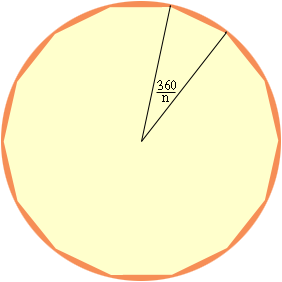Name: Krishna Who is asking: Parent Level of the question: Secondary Question: I have to find out the chord length of a polygon - Tetradecagon ! The Radius of the Circle is 11.5 Cms. The Circle is intersepted by 14 arcs. Then how to find out the chord length? Hi there. If you are trying to find the length of a regular polyhedron with n sides, you can think of these n sides as taking you all the way around a circle of 360 degrees. That means the angle subtended by a single side is 360/n degrees.If you have the radius and n and want to know the length of the side, then you can use the Law of Cosines to solve for it. You have an isoceles triangle with common length equal to the radius and the included angle is 360/n, so: c2 = a2 + b2 - 2abcosC c2 = r2 + r2 - 2r2 cos(360/n) c2 = 2r2 (1-cos(360/n)) Hope this helps, Stephen La Rocque.